# Measuring additive interaction using odds ratios

## Abstract

Interaction measured on the additive scale has been argued to be better correlated with biologic interaction than when measured on the multiplicative scale. Measures of interaction on the additive scale have been developed using risk ratios. However, in studies that use odds ratios as the sole measure of effect, the calculation of these measures of additive interaction is usually performed by directly substituting odds ratios for risk ratios. Yet assessing additive interaction based on replacing risk ratios by odds ratios in formulas that were derived using the former may be erroneous. In this paper, we evaluate the extent to which three measures of additive interaction – the interaction contrast ratio (ICR), the attributable proportion due to interaction (AP), and the synergy index (S), estimated using odds ratios versus using risk ratios differ as the incidence of the outcome of interest increases in the source population and/or as the magnitude of interaction increases. Our analysis shows that the difference between the two depends on the measure of interaction used, the type of interaction present, and the baseline incidence of the outcome. Substituting odds ratios for risk ratios, when calculating measures of additive interaction, may result in misleading conclusions. Of the three measures, AP appears to be the most robust to this direct substitution. Formulas that use stratum specific odds and odds ratios to accurately calculate measures of additive interaction are presented.

## Background

Interaction in epidemiology refers to the extent to which the joint effect of two risk factors on disease differs from the independent effects of each of the factors [1, 2]. The joint effect is the effect of the presence of both factors on disease and the independent effect is the effect of each factor in the absence of the other factor. In terms of their causal effects on the incidence of a disease, two risk factors may act independently or interact thereby augmenting (in case of synergism) or reducing (in case of antagonism) the effect of one another. Epidemiologists speak of two distinct types of interaction, biological interaction and statistical interaction. Statistical interaction is a model-dependent concept . It is considered to be present on a multiplicative scale when the joint effect of risk factors differs from the product of the effects of the individual factors . Statistical interaction is present on the additive scale when the joint effect of risk factors differs from the sum of the effects of the individual factors.

Biological interaction on the other hand, describes a property of causality and refers to the interdependent action of two or more factors to produce or prevent an effect . There are limitations in inferring biological/causal interaction based on statistical assessment of interaction. Frequently investigators are not able to identify all of the underlying pathological processes involving measured or unmeasured intervening variables and their effect on the incidence of disease . However, the concept of biological interaction is important in epidemiology for predicting disease on the basis of an individual's profile for a set of risk factors and for planning interventions at the policy level . The appropriate scale on which to statistically assess interaction in such a way that it reflects biological interaction has been a controversial topic in epidemiology. It has been argued that the assessment of interaction on the additive scale is more indicative of the underlying causal mechanism [3, 5]. Thus some authors have advocated that the statistical assessment of interaction is best done on the additive scale [3, 511].

Additive interaction is measured in epidemiological studies primarily using the difference of risk differences also known as the interaction contrast (IC). In cohort studies, this can easily be accomplished by fitting linear or log-linear risk models. However, IC cannot be estimated from case control studies unless the sampling fractions for cases and controls are known or can be estimated . Alternative measures of interaction on the additive scale have been derived from the IC and are based on risks ratios (RR) (see appendix). These measures are, the relative excess risk due to interaction (RERI) also called the interaction contrast ratio (ICR), the attributable proportion due to interaction (AP), and the synergy index (S) [3, 6, 7, 9]. The ICR is the excess risk due to interaction relative to the risk without exposure. AP refers to the attributable proportion of disease which is due to interaction among persons with both exposures. S is the excess risk from exposure (to both exposures) when there is interaction relative, to the excess risk from exposure (to both exposures) without interaction. These measures can be used to assess additive interaction when the odds ratio estimates the risk ratio. However, it is recognized that odds ratios from case-control studies that are not designed to directly estimate the risk or rate ratio, only approximate the risk ratio well when the outcome is rare and do so poorly when the incidence (average risk) of the outcome of interest is high in the source population [6, 1315]. The more frequent the outcome becomes, the more the odds ratio overestimates the risk ratio when it is more than 1 and underestimates the risk ratio when it is less than 1. In addition, the difference between the odds ratio and the risk ratio also depends on the magnitude of the effect of the risk factor. As the effect of a risk factor increases so does the difference between the odds ratio and the risk ratio.

The implications of interpreting measures of interaction on the multiplicative or additive scale when using odds ratios in place of risk ratios have received little attention in the literature. Previous studies have shown that the differences that could arise from assessing interaction using odds ratios instead of risk ratios could be so important as to warrant their careful consideration in epidemiologic research. Morabia et al noted that using odds ratios instead of risk ratios can result in discrepant results when assessing statistical interaction on the multiplicative scale . Campbell et al further showed that there was a difference in the estimates of interaction assessed both on the additive and multiplicative scale when odds ratios were used in place of risk ratios using ICR . The magnitude of this difference depended both on the baseline risk as well as the magnitude of the joint and independent risk ratios. However, Morabia et al only assessed interaction on the multiplicative scale  and although Campbell et al, assessed interaction on both the additive and multiplicative scale, they only evaluated the performance of ICR and did not include the other measures of interaction, AP and S . In addition, the interaction was assessed within a limited range of effect magnitudes.

All three measures of interaction ICR, AP and S have been used widely in epidemiologic studies . However, there have been no studies that have assessed the validity of AP and S as measures of interaction when odds ratios are used in place of risk ratios. In this paper we assess the extent to which the three measures of interaction ICR, AP and S estimated using risk ratios and odds ratios differ as the incidence of the outcome of interest increases in the source population and/or as the magnitude of interaction increases.

## Analysis

### Notation and measures of additive interaction

Throughout this paper the risk of an outcome under the influence of two independent binary exposure variables is considered. The risk of the outcome (the probability of the outcome given an exposure level) is denoted R while the odds of the outcome (the ratio of the probability that the outcome is observed, to the probability of the outcome not being observed given an exposure level) is denoted O. Risk and odds are represented by Rij and Oij respectively, with i indexing exposure to the first variable, and j indexing exposure to the second variable. The subscripts i and j take values of 0 or 1 in the absence or presence of exposure respectively. Thus R11 stands for the risk of the outcome when doubly exposed, while O10 stands for the odds of the outcome given exposure to the first factor and non-exposure to the second factor. Similarly, using RR and OR to denote risk ratio and odds ratio respectively and using doubly unexposed to both factors as the reference, RR01 stands for the RR comparing the risk of the outcome in subjects exposed to the second factor but not to the first, to the risk in the doubly unexposed (R01/R00) while OR11 stands for the OR comparing the doubly exposed to the doubly unexposed (O11/O00).

Additive interaction is assessed using the three measures proposed by Rothman [3, 9] – the interaction contrast ratio (ICR), the attributable proportion due to interaction (AP) and Rothman's synergy index (S). In this paper, when these are calculated using the RR as suggested by Rothman, they are referred to as ICR, AP and S. However, when they are calculated by substituting ORs for RRs they are referred to as ICRF, APF and SF respectively (F stands for 'false' as these are false measures of ICR, AP and S). Formulas for these measures are given in the appendix.

### Simulations and assumptions

Using SAS statistical software version 9 (by the SAS Institute Inc. Cary, NC, USA) risks for the doubly exposed (R11) and the doubly unexposed (R00) portions of 100,000 scenarios were simulated using a random uniform distribution of risks ranging from 0 to 1. The simulations allowed us, not only to examine a wide range of combinations of the baseline risk and magnitude of interaction, but they also permitted us to describe the shape and magnitude of discrepancy between the interaction measures using RR and OR. To simplify the comparison of the measures of interaction, the effects of each of the exposures were fixed to 2 such that in each cohort RR10 = RR01= 2. Thus to avoid implausible values of R01 and R10 (risk values greater than 1) this analysis was limited to simulated cohorts in which the baseline risks (R00) were less than 0.5. This analysis was also restricted to that of two causative factors such that only cohorts where R01 and R01 were both greater than R00 were included. This did not preclude R11 from being less than R00 and/or R10 (or R01) thus allowing for cohorts in which the risk factors were antagonistic to each other. We also assumed complete assessment of the population such that risks and odds (and consequently risk ratios and odds ratios) were assessed without sampling error. Finally, the absence of any confounders of the effect of each of the factors in all the cohorts was also assumed.

## Results

A similar relationship is noted when additive interaction is assessed using AP. As illustrated in the Figure 2, the difference in the magnitude of APF from AP increases with an increase in the baseline risk. However, this difference only becomes appreciably evident at a baseline risk of approximately 0.15, which is higher than that seen when using ICR. At the baseline risk of 0.05, there is a small difference in the AP and APF values. The AP values of 0, 0.25 and 0.40 have corresponding APF values of 0.03, 0.32 and 0.49 respectively. However, at the baseline risk of 0.15, the differences in magnitude are noticeable as the corresponding APF values are 0.06, 0.55 and 0.77 respectively. Furthermore, the differences in the estimates of AP and APF are not as pronounced when the interaction is more than additive, as was the case when using ICR to measure interaction. However, as opposed to using ICR, the difference is more pronounced when the interaction is less than additive. When the value of AP is -1, the difference in the estimates of AP and APF are not substantial even at baseline risk levels of 0.25 where the value of APF is -1.8. However, when the magnitude of the less than additive interaction between the two risk factors increases and the AP value is -5, the difference in the estimates of AP and APF become very marked even at a lower baseline risk of approximately 0.05 such that the value of APF is 6.4.

As illustrated in Figure 3, a similar pattern is also observed when assessing the presence of additive interaction using S. The discrepancy in the values of S and SF increases when the baseline risk increases and the magnitude of interaction increases. When there is exact additivity, indicating no interaction on the additive scale, S has a value of 1. However, there is divergence of the values of SF from S, in the scenario where we would have expected exact additivity if risk ratios had been used. This is appreciably evident when the baseline risk is approximately 0.25 where the value of SF is 1.5 instead of 1. This noticeable difference is at a much higher baseline risk than that seen when the two previous measures of interaction, ICR and AP were used. As was noted in the case of ICR, the differences in the estimates of S and SF become more evident at lower levels of baseline risk when the is interaction is greater than additive. For example, when the interaction is more than additive and more than multiplicative, the difference in the two estimates is appreciable even at baseline risks as low as 0.05 such that the value of SF is 4.2, instead of an S value 3. Because of the predetermined risk ratio of 2 for both risk factors, for our simulations the lowest value that S can have is -0.5. When there is less than additive interaction the discrepancy in the values of S and SF decreases as the value of S approaches 1.

The effect of calculating the three interaction measures using odds ratios instead of risk ratios is further illustrated in Figure 4. The size of the bubbles represents the magnitude of the difference between the interaction measures using odds ratios and risk ratios. The lines represent the scenario when there is exact additivity. Panel 4a (left upper corner) represents the absolute difference in the values of ICR and ICRF. It can be noted that the difference in these estimates increases with an increase in the baseline risk. In addition, the difference is more evident in the presence of interaction that is more than additive compared to the other types of interaction as indicated by the size of the bubbles.

Panel 4b (right upper corner) is a representation of the estimates of interaction calculated using AP and APF. As was the case for ICR/ICRF, the differences in the absolute values increase as the baseline risk increases. However, in this instance the increase in the discrepancy between AP and APF is more gradual in contrast to ICR/ICRF, and the difference is more prominent when the interaction is less than additive. Panel 4c (bottom left corner) represents the absolute difference between S and SF and Panel 4d (bottom right corner) represents the log difference between S and SF. The pattern is very similar to that seen when the presence of interaction was assessed using ICR/ICRF. The difference increases as the baseline risk increases and it is more prominent when there is interaction that is more than additive.

## Discussion

Morabia et al. previously described a similar discrepancy between the OR and RR when assessing interaction but this was limited to interaction on the multiplicative scale . Campbell et al also reported similar discrepancies both on the multiplicative scale and on the additive scale using the ICR . Although most authors tend to assess interaction on the multiplicative scale when using ORs, particularly in the context of case-control study designs and/or logistic regression analysis [2224, 26], in this paper we only evaluated the assessment of interaction on the additive scale. In simulations using individual level etiologic models (such as the Potential Outcomes Model and the Sufficient and Component Cause Model), the additive scale appeared to be a better indicator of the relative frequency of antagonistic or synergistic interactions in the study population [3. 5]. Thus the additive scale has been argued to be the scale of choice to assess interaction.

The three measures ICR, AP and S were developed independently. ICR and AP were derived directly from IC which is the primary measure of additive interaction as illustrated in the formulae in the appendix. ICR expresses IC as a proportion to the baseline risk (R00) and AP expresses IC as a proportion of the joint effect of the two risk factors (R11). S is the ratio of the observed and expected effects of the difference between the joint effect and the baseline risk. Of the three measures of interaction on the additive scale (ICR, AP, and S), the ICR appears to be most frequently utilized [3, 25, 27]. However, ICR may not be the best measure to use for assessing interaction in all circumstances. Our study suggests that AP is more robust to directly substituting ORs in place of RRs. It is less affected, compared to ICR and S, by changes in the type of interaction or baseline risk when substituting risk ratios by odds ratios. Furthermore, in the analysis of additive interaction while adjusting for confounding (using additional covariates in regression models), S has been shown to be the most reliable as it does not vary across strata of the additional covariates . We suggest that all three measures be used when assessing additive interaction and that any discrepancies between the three should be resolved by a careful consideration of the individual risk factors being evaluated, the baseline risk of the outcome, as well as the presence or not of confounders.

Extensive work has been done to accommodate odds ratios in epidemiologic studies . However, to the best of our knowledge, these do not address the measurement of additive interaction when left only with odds ratios as measures of effect. Campbell et al provide a formula that shows how a measure of multiplicative interaction based on risk ratios can be estimated using odds ratios and baseline risk . We have derived formulas to calculate the three additive interaction measures using odds ratios. The formulas presented in the appendix could be used to more accurately calculate the three additive measures of interaction, similar to what would have been obtained, had the risk ratio been used. Odds for each level defined by combinations of exposure and covariate can be estimated using parameters from a logistic regression. The formulas could easily be implemented using a spreadsheet (see Additional File 1).

## Conclusion

Substituting ORs for RRs, when calculating measures of additive interaction (ICR, AP and S), may result in misleading conclusions. The magnitude of the discrepancy in the measures of interaction increases with an increase in the baseline incidence of the outcome and depends on the measure of interaction used as well as the type of interaction. We recommend that formulas modified for direct use of odds and odds ratios be employed for more accurate assessment of additive interaction.

## Appendix

### I Measures of additive interaction

The interaction contrast (IC) which is the basic measure of additive interaction is applicable when the risks for each stratum defined by 2 variables are available. It is given by:

IC = R11 - R10 - R01 + R00    Eq. 1

However when only the risk ratios (and not the risks for each level defined by exposure-covariate combinations) are available, the following extensions of IC can be used to evaluate additive interaction [3, 9]

IC = R11 - R10 - R01 + R00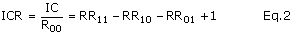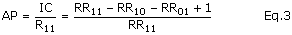ICR and AP thus have a null value of 0 while S has a null value of 1. With some study designs, such as cross-sectional studies or case-control studies, the only measure of effect that can be obtained is the odds ratio, OR. To evaluate interaction on the additive scale some authors have simply substituted OR for RR in equations 24. We refer to ICR, AP and S calculated using the OR as ICRF, APF, and SF respectively. If the OR is not a good estimate of the RR then ICRF, APF and SF may not be good estimates of ICR, AP and S respectively.

To correctly calculate ICR, AP and S, from studies in which only the OR is available one simply needs to calculate the RR based on the OR and substitute it in equations 24 (instead of directly substituting OR for RR).

To calculate the RR using the OR, consider a single binary exposure scenario: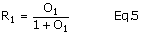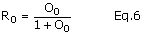Thus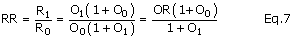Extending equation 7 to the two binary exposure variable scenario then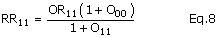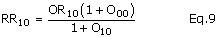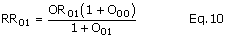Substituting equations 810in equations 24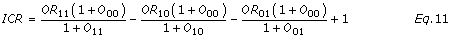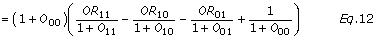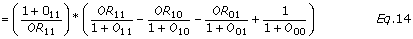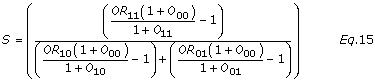Thus, to more accurately calculate ICR, AP and S, the odds of the outcome for each level defined by combinations of the 2 covariates being evaluated for interaction, need to be known.

These odds can be estimated using parameters from a logistic regression and sampling probabilities (when needed) as follows { pp 416–422}:Where

k is the number of exposure levels defined by combinations of covariates (for example for 2 binary covariates, k = 4);

Wj are mutually exclusive indicator variables for each of the k-l exposure levels (excluding a referent level);

βj are the estimated log odds ratios comparing each index j level to the referent level;

α* is the estimated intercept parameter from the logistic regression;

f and g are case and control sampling probabilities respectively.

Note that equation 16 is based on the fact that in case-control studies, the intercept from a logistic regression model is the log of the odds of the outcome inflated by the log of the ratio of case to control sampling probabilities .

In cohort studies, cross sectional studies, or case control studies with complete ascertainment of both cases and controls, in which analysts use logistic regression, f = g = 1, and equation 16 reduces to equation 17Equations 12, 14and 15 can be easily implemented using a spreadsheet (see Additional File 1).

### II. SAS code

data one simulate;

do sample = 1 to 100000;

r00 = round((0.00001 + ranuni(11)),.01);

r11 = round ((0.00001 + ranuni(14)), 0.01);

r01 = .;

if r00<0.5 then r01 = 2*r00;

r10 = .;

if r00<0.5 then r10 = 2*r00;

rr11 = r11/r00;

rr10 = r10/r00;

rr01 = r01/r00;

or11 = (r11*(1-r00))/(r00*(1-r11));

or01 = (r01*(1-r00))/(r00*(1-r01));

or10 = (r10*(1-r00))/(r00*(1-r10));

icr = round(((r11/r00)-(r10/r00)-(r01/r00)+1), .01);

ap = round(((r11/r00)-(r01/r00)-(r10/r00)+1)/(r11/r00), .01);

s = round(((rr11-1)/(rr10 + rr01 -2)), .01);

icrf = round((or11- or10- or01 + 1), .01);

apf = round((or11-or01-or10+1)/or11, .01);

sf = round((or11-1)/(or10 + or01 -2), .01);

icrdif = abs(icrf-icr);

apdif = abs(apf-ap);

sdif = abs(sf-s);

logsdif = abs(log(sf) – log(s));

output simulate;

end;

run;

proc sort data = simulate; by r00 ; run;

axis1 order = -10 to 20 by 5 label = (a = 90 r = 0 'ICRF' h = 2);

axis2 order = 0 to 0.4 by 0.1;

procgplot data = simulate ; where icr in (-2.5,-1.5, 0,0.5,1, 2, 4,);

symbol1 i = spline v = star h = 0.5 c = black;

symbol2 i = spline v = plus h = 0.5 c = red;

symbol3 i = spline v = circle h = 0.5 c = blue;

symbol4 i = spline v = diamond h = 0.5 c = green;

symbol5 i = spline v = square h = 0.5 c = violet;

symbol6 i = spline v = triangle h = 0.5 c = indigo;

symbol7 i = spline v = dot h = 0.5 c = black;

plot icrf*r00 = icr/haxis = axis2 vaxis = axis1 nolegend;

run;quit;

axis1 order = -10 to 3 by 1 label = (a = 90 r = 0 'APF' h = 2);

axis2 order = 0 to 0.4 by 0.1;

proc gplot data = simulate ;

where icr in (-2.5,-1.5, 0, 0.5,1, 2, 4,);

symbol1 i = spline v = star h = 0.5 c = black;

symbol2 i = spline v = plus h = 0.5 c = red;

symbol3 i = spline v = circle h = 0.5 c = blue;

symbol4 i = spline v = diamond h = 0.5 c = green;

symbol5 i = spline v = square h = 0.5 c = violet;

symbol6 i = spline v = triangle h = 0.5 c = indigo;

symbol7 i = spline v = dot h = 0.5 c = black;

plot apf*r00 = ap/haxis = axis2 vaxis = axis1 nolegend;

run;quit;

axis1 order = -5 to 15 by 1 label = (a = 90 r = 0 h = 1 'SF' h = 2);

axis2 order = 0 to 0.4 by 0.1;

proc gplot data = simulate ;

where icr in (-2.5,-1.5, 0, 0.5,1, 2, 4,);

symbol1 i = spline v = star h = 0.5 c = black;

symbol2 i = spline v = plus h = 0.5 c = red;

symbol3 i = spline v = circle h = 0.5 c = blue;

symbol4 i = spline v = diamond h = 0.5 c = green;

symbol5 i = spline v = square h = 0.5 c = violet;

symbol6 i = spline v = triangle h = 0.5 c = indigo;

symbol7 i = spline v = dot h = 0.5 c = black;

plot sf*r00 = s/haxis = axis2 vaxis = axis1 nolegend;

run;quit;

*bubble plots;

data anno2;/*this superimposes a line where there is exact additivity ie icr = 0, rr11 = 3 */function = 'move'; xsys = '1'; ysys = '1'; x = 0; y = 0; output;

function = 'draw'; xsys = '1'; ysys = '1'; color = 'red'; x = (100/3)*2; y = 100; output;

run;

axis1 order = 0 to 1 by .1;

axis2 order = 0 to 0.4 by 0.1;

proc gplot data = simulate ;

where icr in (-3, -2, -1, 0, 1,3, 5);

bubble r11*r00 = icrdif/anno = anno2 haxis = axis2 vaxis = axis1 bsize = 100 bcolor = blue;

bubble r11*r00 = apdif/anno = anno2 haxis = axis2 vaxis = axis1 bsize = 100 bcolor = blue;

bubble r11*r00 = sdif/anno = anno2 haxis = axis2 vaxis = axis1 bsize = 100 bcolor = blue;

bubble r11*r00 = logsdif/anno = anno2 haxis = axis2 vaxis = axis1 bsize = 100 bcolor = blue;

run;quit;

## Abbreviations

R:

Risk

O:

Odds

RR:

Risk ratio

OR:

Odds ratio

RERI:

Relative excess risk due to interaction

ICR:

Interaction contrast ratio

AP:

Attributable proportion due to Interaction

S:

Rothman's synergy index

ICRF:

Interaction contrast ratio calculated by substituting odds ratios for risk ratios

APF:

Attributable proportion due to interaction calculated by substituting odds ratios for risk ratios

SF:

Rothman's synergy index calculated by substituting odds ratios for risk ratios

## References

1. Miettinen O: Confounding and effect-modification. Am J Epidemiol 1974, 100:350–353.

2. Ahlbom A, Alfredsson L: Interaction: A word with two meanings creates confusion. Eur J Epidemiol 2005, 20:563–564.

3. Rothman KJ, Greenland S, Ed: Modern epidemiology Lippncott Williams and Wilkins, Philadelphia, PA 1998.

4. Thompson WD: Effect modification and the limits of biological inference from epidemiologic data. J Clin Epidemiol 1991, 44:221–232.

5. Greenland S, Poole C: Invariants and noninvariants in the concept of interdependent effects. Scand J Work Environ Health 1988, 14:125–129.

6. Rothman KJ: Synergy and antagonism in cause-effect relationships. Am J Epidemiol 1974, 99:385–388.

7. Rothman KJ: The estimation of synergy or antagonism. Am J Epidemiol 1976, 103:506–511.

8. Rothman KJ, Greenland S, Walker AM: Concepts of interaction. Am J Epidemiol 1980, 112:467–470.

9. Rothman KJ, Ed: Modern epidemiology Little, Brown and Company, Boston, MA 1986.

10. Darroch J: Biologic synergism and parallelism. Am J Epidemiol 1997, 145:661–668.

11. Koopman JS, Weed DL: Epigenesis theory: A mathematical model relating causal concepts of pathogenesis in individuals to disease patterns in populations. Am J Epidemiol 1990, 132:366–390.

12. Skrondal A: Interaction as departure from additivity in case-control studies: A cautionary note. Am J Epidemiol 2003, 158:251–258.

13. Rodrigues L, Kirkwood BR: Case-control designs in the study of common diseases: Updates on the demise of the rare disease assumption and the choice of sampling scheme for controls. Int J Epidemiol 1990, 19:205–213.

14. Zocchetti C, Consonni D, Bertazzi PA: Relationship between prevalence rate ratios and odds ratios in cross-sectional studies. Int J Epidemiol 1997, 26:220–223.

15. Davies HT, Crombie IK, Tavakoli M: When can odds ratios mislead? BMJ 1998, 316:989–991.

16. Morabia A, Ten Have T, Landis JR: Interaction fallacy. J Clin Epidemiol 1997, 50:809–812.

17. Campbell UB, Gatto NM, Schwartz S: Distributional interaction: Interpretational problems when using incidence odds ratios to assess interaction. Epidemiol Perspect Innov 2005, 2:1.

18. Moceri VM, Kukull WA, Emanual I, van Belle G, Starr JR, Schellenberg GD, McCormick WC, Bowen JD, Teri L, Larson EB: Using census data and birth certificates to reconstruct the early-life socioeconomic environment and the relation to the development of alzheimer's disease. Epidemiology 2001, 12:383–389.

19. Solovieva S, Lohiniva J, Leino-Arjas P, Raininko R, Luoma K, Ala-Kokko L, Riihimaki H: COL9A3 gene polymorphism and obesity in intervertebral disc degeneration of the lumbar spine: Evidence of gene-environment interaction. Spine 2002, 27:2691–2696.

20. Padyukov L, Silva C, Stolt P, Alfredsson L, Klareskog L: A gene-environment interaction between smoking and shared epitope genes in HLA-DR provides a high risk of seropositive rheumatoid arthritis. Arthritis Rheum 2004, 50:3085–3092.

21. Olshan AF, Weissler MC, Watson MA, Bell DA: Risk of head and neck cancer and the alcohol dehydrogenase 3 genotype. Carcinogenesis 2001, 22:57–61.

22. Donato F, Tagger A, Gelatti U, Parrinello G, Boffetta P, Albertini A, Decarli A, Trevisi P, Ribero ML, Martelli C, et al.: Alcohol and hepatocellular carcinoma: The effect of lifetime intake and hepatitis virus infections in men and women. Am J Epidemiol 2002, 155:323–331.

23. Garcia Rodriguez LA, Varas-Lorenzo C, Maguire A, Gonzalez-Perez A: Nonsteroidal antiinflammatory drugs and the risk of myocardial infarction in the general population. Circulation 2004, 109:3000–3006.

24. Green RM, Custovic A, Sanderson G, Hunter J, Johnston SL, Woodcock A: Synergism between allergens and viruses and risk of hospital admission with asthma: Case-control study. BMJ 2002, 324:763.

25. Rauscher GH, Sandler DP, Poole C, Pankow J, Shore D, Bloomfield CD, Olshan AF: Is family history of breast cancer a marker of susceptibility to exposures in the incidence of de novo adult acute leukemia? Cancer Epidemiol Biomarkers Prev 2003, 12:289–294.

26. Lee CH, Lee JM, Wu DC, Hsu HK, Kao EL, Huang HL, Wang TN, Huang MC, Wu MT: Independent and combined effects of alcohol intake, tobacco smoking and betel quid chewing on the risk of esophageal cancer in taiwan. Int J Cancer 2005, 113:475–482.

27. Hauser R, Williams P, Altshul L, Calafat AM: Evidence of interaction between polychlorinated biphenyls and phthalates in relation to human sperm motility. Environ Health Perspect 2005, 113:425–430.

28. Greenland S: Model-based estimation of relative risks and other epidemiologic measures in studies of common outcomes and in case-control studies. Am J Epidemiol 2004, 160:301–305.

## Acknowledgements

We are grateful to Drs Jay Kaufman and William C Miller for their very helpful comments. We also thank Drs Ulka Campbell, Nicole Gatto, George Maldonaldo and Sander Greenland for a very insightful review. JA and LK are both sponsored by the Fogarty AITRP grant to the University of North Carolina at Chapel Hill.

## Author information

Authors

### Corresponding author

Correspondence to Julius Atashili.

### Competing interests

The author(s) declare that they have no competing interests.

### Authors' contributions

LK conceived the research question. LK and JA designed and conducted the analysis. Both authors wrote the manuscript.

## Rights and permissions

Reprints and Permissions

Kalilani, L., Atashili, J. Measuring additive interaction using odds ratios. Epidemiol Perspect Innov 3, 5 (2006). https://doi.org/10.1186/1742-5573-3-5